Introduction to Lagged Weighted Quantile Sum Regression

library(lwqs)

Introduction

The lWQS package provides a convenient wrapper based on the gWQS and gamm4 packages to implement lagged weighted quantile sum (lWQS) regression1. This provides an approach for the estimation of time-varying mixture effects, particularly in contexts of environmental medicine and public health. The general premise of the procedure is to integrate the generalized weighted quantile sum regression (WQS) algorithm2 in a longitudinal context with repeated measures. To achieve this, WQS is applied to estimate mixture effects at specific time points; the mixture indices derived from WQS are then used to construct a longitudinal model, consistent with the reverse distributed lag approach outlined by Chen et al3. The package is currently in development and users should expect syntax and functionality to evolve over time.

General Usage, Requirements, and Data Structures

Lagged weighted quantile sum regression is used to link fixed outcome data to the mixed effects of multiple time-varying predictors. Repeated measures should be organized in “long” format, with each row representing a successive observation per subject. For purposes of estimating confidence intervals the package assumes a z-scored outcome distribution.

The package includes a simulated data set (lwqs_data) that illustrates the intended data structure for use of this package. Data can be attached and viewed as in the below:

library(lwqs)
data(lwqs_data)

The simulated data are structured as in below snippet (use head(lwqs_data) to reconstruct) from the lwqs_data. Note that each simulated person - identified by the “ID” variable - has a single fixed outcome, identified as “outcome”. The outcome variable was simulated from a standardized (z-score) Gaussian distribution. A simulated binary covariate, “sex”, was also generated from a binomial distribution. As well, each individual has 5 simulated predictor variables (pred1-pred5) which are measured at 30 distinct time points (“time”). In an environmental epidemiological study design, this might correspond to 5 measurements of exposure collected at each month post birth for a total of 30 months; and, our goal is to identify the developmental window at which the mixture of exposures relates to our health outcome, while adjusting for a covariate (s) such as sex.

Note the provided data are “balanced” in the sense that all participants are sampled at every sampling interval. In practice, with data providing incomplete or unbalanced coverage, it may be necessary to prune, aggregate, or otherwise manipulate data to ensure reliable estimates. If only a handful of subjects were observed at a given sampling interval, for example, it will be difficult to generate reliable estimates; or, if a given sampling interval only includes 1 level of some key covariate (e.g., only females are sampled at time point 3 in a model with sex as a covariate) this may compromise model convergence and/or effect estimation.

#>    ID sex time        out      pred1      pred2      pred3      pred4      pred5
#> 1   1   1    1 -0.8322414 -0.2062299 1.30295010 -1.3870911  0.1607504 -0.1757468
#> 12  1   1    2 -0.8322414 -0.0941091 0.42957397 -0.4552804  0.3642088 -1.1884153
#> 23  1   1    3 -0.8322414  2.9357351 0.01995526  0.7725593  0.8464955 -1.4320769
#> 25  1   1    4 -0.8322414 -1.3437874 0.86728598  0.7679771  0.1602173 -0.7590517
#> 26  1   1    5 -0.8322414  0.7142656 0.30884399  0.7302721  0.1933808  0.3498828
#> 27  1   1    6 -0.8322414  0.6871639 1.26009045  0.9717983 -0.0763416  0.6857857

Simulation Parameters

Simulated data were generated with autoregressive models to approximate expected patterns of within-subject correlation. As well, each predictor was simulated with a time-varying association with the health oucome.

Predictors 1 and 2 have no association with the outcome at time points 1-10 and 21-30; but, at time points 11-20 have either a strong positive association with the outcome (predictor 1) or a weak positive association with the outcome (predictor 2).

Likewise, predictors 3 and 4 have no association with the outcome in months following time point 10, but from time 1-10 have either a weak (predictor 3) or strong (predictor 4) negative association with the outcome.

Predictor 5, unlike the others, has no association with the outcome at all.

The below plot illustrates these parameters. On the y-axis the simulated associations between each predictor and the outcome are shown, plotted against the change in these parameters over time (x-axis). Beta 1, beta 2, etc, refer to the effects associated with predictor 1, predictor 2, etc.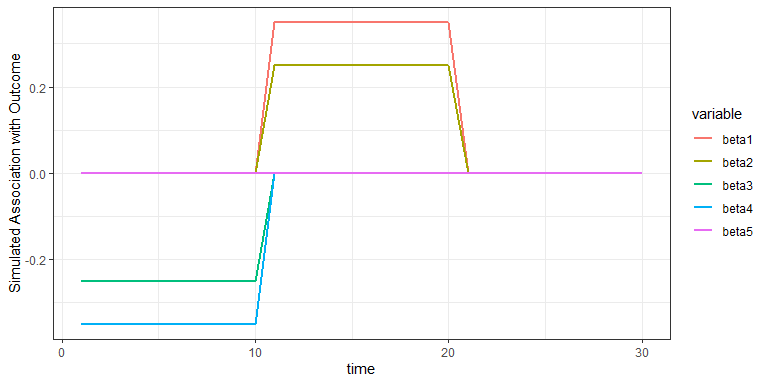Application of lWQS

With respect to the effects summarized above, the lwqs algorithm should identify a negative mixture, comprised of predictor 3 (weak effect) and predictor 4 (strong effect) from times 1-10; and, likewise, a positive mixture, comprised of predictor 1 (strong) and predictor 2 (weak) from time points 11-20. There should be no significant mixture effects from times 21-30.

To dissect the counteracting effects of mixtures acting in opposing directions, the lWQS algorithm leverages the usage of constraints implemented in the weighted quantile regression procedure (see gWQS package for details). In practice this means the algorithm is run twice in succession; first, examining mixture effects that may relate positively to the outcome of interest, and then again to examine negative associations.

Note that lwqs is generally a computationally-intensive procedure involving multiple ensemble steps. For example, the estimation of a model across 30 time points, with 50 bootstraps at each time point, will involve more than 1500 discrete models. Patience is a virtue in this pursuit.

Identifying mixture effects positively associated with outcomes

The below code is used to implement the lWQS algorithm.

First, it is neccesary to identify the time-varying predictors that will comprise the mixture. In our simulation data, these are pred1-pred5, which correspond to columns 5-9 of lwqs_data. In the below code we save these to an index called “mixvars”

Next, we specify the call to the lwqs algorithm. Each parameter will be explained as follows, but in general this involves specifying the identity of a few key variables (ID, time, and outcome variable names), and specifying the desired parameters for the mixture (wqs_parms). Each parameter is identified in the accompanying comments. For now we will ignore covariates. Parameters specific to the longitudinal component of the model (rDLM_parms) are automatically passed to gamm4. For users that may wish to modify these parameters for covariate adjustment, model selection, etc, we recommend the use of the extract_function() procedure explained subsequently.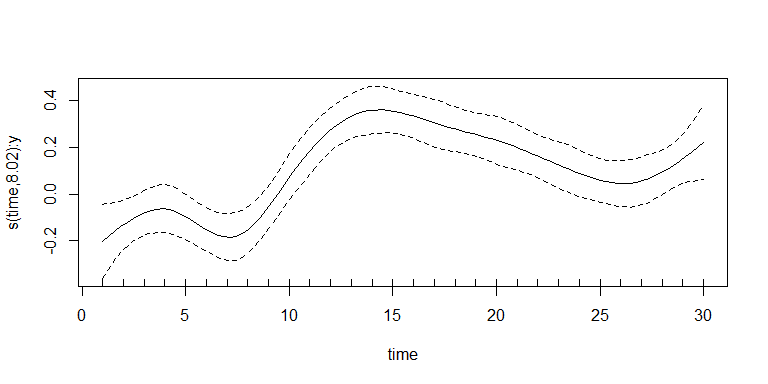#> geom_smooth() using formula 'y ~ x'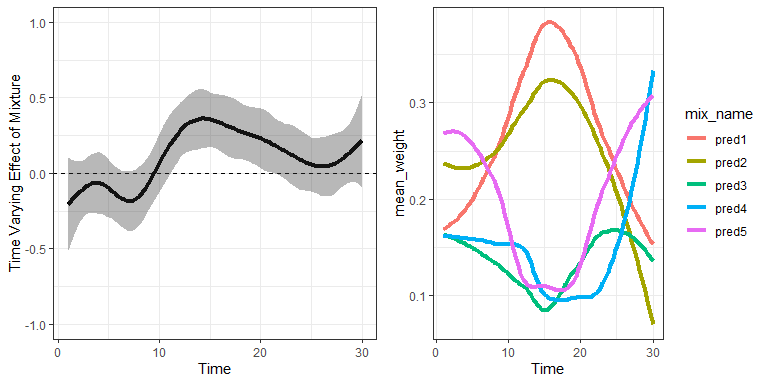By default, the lwqs function will generate two plots to summarize the model output, as in the above. In the left panel, the time-varying effect estimate associated with the exposure mixture is shown. Note that the confidence intervals on this estimate overlap zero except for the interval from ~11-20. This would be interpreted as the “critical window” in which our simulated exposures positively relate to the outcome of interest. In the right plot, the time-varying weights estimated for each predictor contributing to the mixture are shown. We would focus our interpretation on time points where the mixture is significant, i.e. where the confidence intervals at left depart from zero. At those time points, the mixture is dominated by predictors 1 and 2, with predictor 1 contributing the most, consistent with the parameters of the simulation.

Note: in the code provided the “b” parameter is set to 5, which indicates 5 bootstrap samples are used at time point in the ensemble estimation step. This is done purely to create a fast-running example; in practice, the number of bootstraps must be sufficient that one can have confidence of an accurate aggregate estimate This parameter should be tuned with use but in general between 30-100 bootstraps may be sufficient for the standard ensemble, depending on processing limitations.

Identifying mixture effects negatively associated with outcomes

The procedure for testing for mixture effects in opposing directions is identical except for the reversal of one parameter. To test for negative associations with an outcome, alter the “b1_pos” parameter in the call to the gWQS function to be set to “FALSE”, as in the below. This will specificy the estimation of mixtures constrained toward negative associations.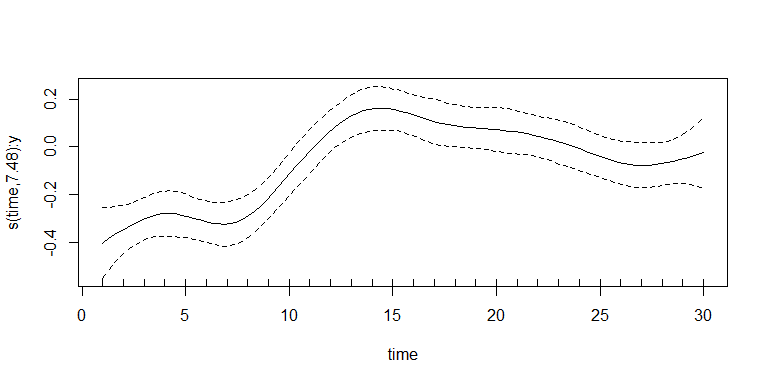#> geom_smooth() using formula 'y ~ x'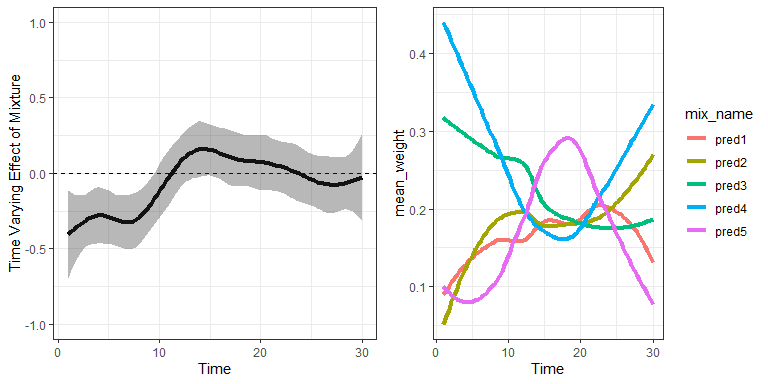As in the prior example, the lwqs function automatically generates two plots to summarize model estimates. At left, the time-varying mixture effect estimates depart from the zero boundary line from time points 1-10, indicating that within this window the simulated predictors are negatively associated with the outcome. At right, we see the weights associated with the mixture in this window are dominated by predictors 3 and 4, which, as per the simulation parameters, exert a moderate and strongly negative association with the outcome.

The models estimated thus far have considered the time-varying association of 5 predictors with a health outcome, but have not been adjusted for covariates. The lwqs algorithm can be adjusted for covariates at two levels; either in the estimation of mixture effects at each sampling interval, or in the estimation of time-varying effects. Users can choose to apply either, neither, or both strategies.

Adjustment for covariates in mixture estimation

Adjustment for covariates at the level of mixture estimation at each sampling interval can be done by modifying the gWQS parameter used in the lwqs function, as below. When specifying the call to the formula used in estimating each interval-specific mixture, simply add the covariate terms to the model formula.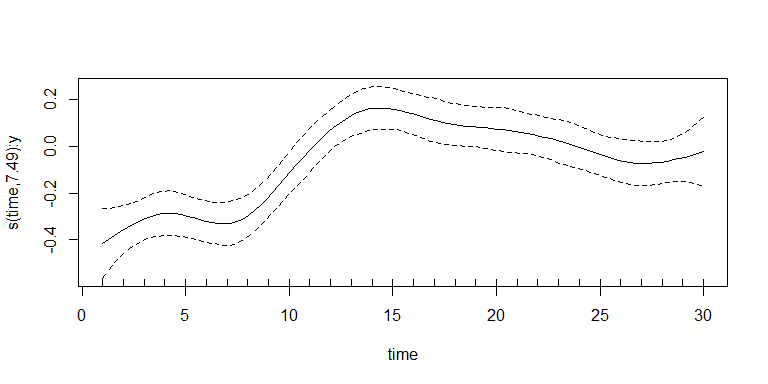#> geom_smooth() using formula 'y ~ x'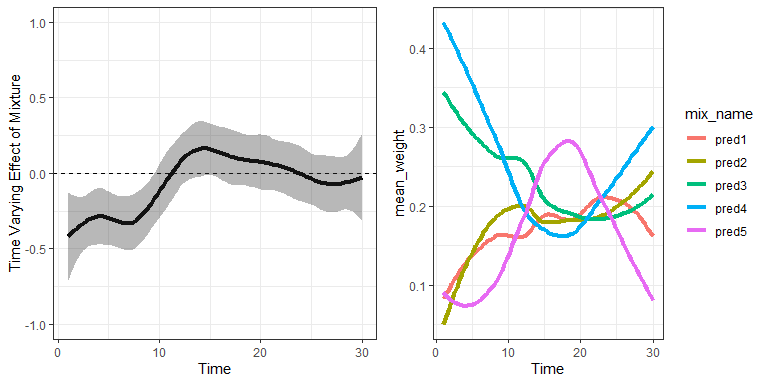Interpretation of the covariate-adjusted model is otherwise identical to previous models; that is, the mixture effect estimates (left panel) indicate a significant negative association with the outcome from time 1-10, and this is driven by predictors 3 and 4 (right panel). The key difference is that in this model those estimates are adjusted for sex.

To evaluate the time-specific significance of each covariate, one could use output stored in the saved model to evaluate parameter estimates for WQS models constructed at each time point. The below code, for example, provides a model summary at time point 1.

Adjustment for covariates in longitudinal model estimation

It may also be advantageous to consider how covariates modify the estimation of time-varying effects. The default lwqs model does not provide this, but an additional function, extract_mixture, can be used to extract the time-varying mixture estimated during the lwqs procedure, at which point it can be used as an input to the gamm4 procedure to allow for covariate adjustment. As an example, the previous model implemented (saved as negcovmod) has implemented an lwqs with adjustment, at the level of the mixture, for sex. Here we use the extract_mixture function to pull the time-varying mixture estimated in this model:

Next, we will merge the covariate data from our original dataset, and input this in a gamm4 model.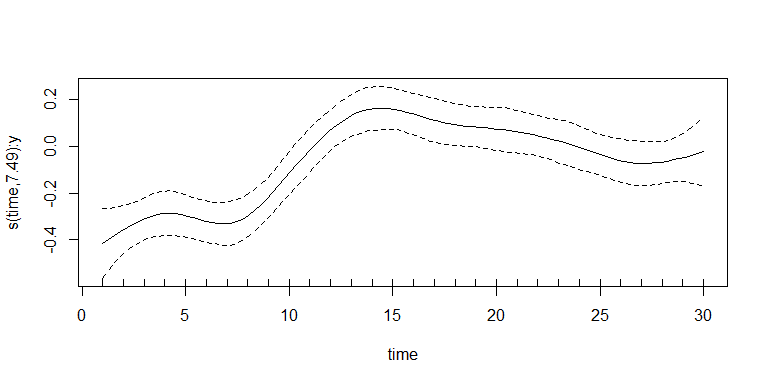This will yield a gamm4 object, from which corresponding plots and parameter estimates can be extracted (see package gamm4 for details). Importantly, note that the error intervals provided in gamm4 are expressed in standard error, rather than 95% confidence intervals. If the latter are of interest toward the research question, these should be expressed with respect to a appropriate critical value, e.g. multiplied by 1.96, assuming a standardized distribution. This is done automatically with the lwqs function, which assumes a z-scored outcome distribution. Effect estimates and corresponding SEs can be extracted from the gamm4 object.

Note as well that the construction of customized gamm4 models to capture mixture effects allows tremendous flexibility in the selection of basis functions, knot intervals, estimation procedures, random effects, and related parameters; these choices may be critical in extending lwqs to contexts such as twin studies, modeling time-varying covariates, and/or focusing on critical intervals. Care should be taken in model selection procedures to ensure appropriate model specification.